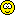Original Post
Junior Member
Black Belt
Join Date: Apr 2011
Posts: 13
Hello everyone! ^.^
My name is Retrou and I love to bet. I made an application to help myself with calculating earnings from bets.

this app can show you:
-House rake
-Winner earnings
-Most profitable bet
-When you reach max profit

Unfortunately you have to write the total ammount of bets, the app is very primitive itself.

You can download it freeware, I hope somebody will find this application usefullSource code code:
``````int main()
{

int a = 0;
for (int i = 1; i == 1; i++)
{
cout << "Welcome to TC bet calculator. \n";
if (a == 0)
{
double  x2, y1, y2;
cout << "1st player total bet TC ammount:";
cin >> y1;
cout << "2nd player total bet TC ammount:";
cin >> y2;
cout << "\t\t\t1st player wins:\n";
cout << "House rake/winner earnings:\t\t" << 0.1 * y2;
cout << "\nBets:\t\t\t";
for (int c = 100; c < 1000; c = c + 100)
{
if ((0.8*y2)*(c / (y1 + c)) >= 5 * c)
cout << "\n" << c << ":\t\t\t\t\t" << c*5 << "\treached max profit";
else
cout << "\n" << c << ":\t\t\t\t\t" << (0.8*y2)*(c / (y1 + c));
}
for (int d = 1000; d < 5000; d = d + 1000)
{
if ((0.8*y2)*(d / (y1 + d)) >= 5 * d)
cout << "\n" << d << ":\t\t\t\t\t" << d * 5 << "\treached max profit";
else
cout << "\n" << d << ":\t\t\t\t\t" << (0.8*y2)*(d / (y1 + d));
}
for (int j = 5000; j < 105000; j = j + 5000)
{
if ((0.8*y2)*(j / (y1 + j)) >= 5 * j)
cout << "\n" << j << ":\t\t\t\t\t" << j * 5 << "\treached max profit";
else
cout << "\n" << j << ":\t\t\t\t\t" << (0.8*y2)*(j / (y1 + j));
}
cout << "\n\t\t\t2nd player wins:\n";
cout << "House rake/winner earnings:\t\t" << 0.1 * y1;
cout << "\nBets:\t\t\t";
for (int q = 100; q < 1000; q = q + 100)
{
if ((0.8*y1)*(q / (y2 + q)) >= 5 * q)
cout << "\n" << q << ":\t\t\t\t\t" << q * 5 << "\treached max profit";
else
cout << "\n" << q << ":\t\t\t\t\t" << (0.8*y1)*(q / (y2 + q));
}
for (int w = 1000; w < 5000; w = w + 1000)
{
if ((0.8*y1)*(w / (y2 + w)) >= 5 * w)
cout << "\n" << w << ":\t\t\t\t\t" << w * 5 << "\treached max profit";
else
cout << "\n" << w << ":\t\t\t\t\t" << (0.8*y1)*(w / (y2 + w));
}
for (int r = 5000; r < 105000; r = r + 5000)
{
if ((0.8*y1)*(r / (y2 + r)) >= 5 * r)
cout << "\n" << r << ":\t\t\t\t\t" << r * 5 << "\treached max profit";
else
cout << "\n" << r << ":\t\t\t\t\t" << (0.8*y1)*(r / (y2 + r));
}
cout << "\n\ndo you want to proceed? (type 0 to continue)\n:";
cin >> i;
}
else;
}

return 0;
}``````

If you have some questions or suggestions for the app, please make a comment. I will try to do my best!

If you want to support me or my content, send some TCSee ya in the betting room!

p.s. dont worry its not trojan.
p.p.s. dont laugh at the code, im a begginer.
Last edited by Retrou; Mar 28, 2018 at 12:24 AM. Reason: adding source code
Belt
Join Date: Jun 2009
Posts: 9,101
Hi! I've moved this thread to the correct place. However, please still add source code if it's not done so in your download link.
I'm Brazilian, i'm alredy fucked every day i wake up

that's some nice C++ there, could use some cleaning up but overall nice.
feel free to join my server in my signature if you ever need help with programming.

might use this here and there, only thing i'd change is maybe put the calcuation stuff in a function."Thinking will not overcome fear but action will." - Clement Stone
Unbound | Super Moderator | ToriAgent | Clan Squad
Junior Member
Black Belt
Join Date: Apr 2011
Posts: 13
Thank you for your comment! I know there is a lot of mess (for example "a" is useless, it was used in different part and never removed) and the main is too long, but the point was to write something working really fast (30 minutes). Im thinking about some java aplets which can operate on data gathered from toribash. Topic is pretty hot for me, i have to pass my exams.
Thank you for your comment! I know there is a lot of mess (for example "a" is useless, it was used in different part and never removed) and the main is too long, but the point was to write something working really fast (30 minutes). Im thinking about some java aplets which can operate on data gathered from toribash. Topic is pretty hot for me, i have to pass my exams.

Once again, if ya ever need help my server has some helpful people
and i mainly focus on optimizing/removing useless stuff once i get functionality down.

Another thing i'd change is instead of using \n in strings to create a new line, do cpp code:
``<< endl;``
after the string
Last edited by hanna; Mar 28, 2018 at 02:54 AM."Thinking will not overcome fear but action will." - Clement Stone
Unbound | Super Moderator | ToriAgent | Clan Squad